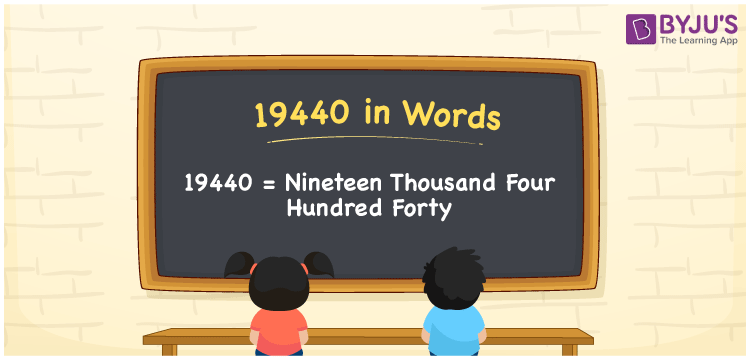# 19440 in Words

We can write 19440 in words as Nineteen thousand four hundred forty. If you deposited an amount of Rs. 19440 in the bank account, then you can say, “I deposited an amount of Nineteen thousand four hundred forty rupees in the bank account”. Therefore, we use the word form of the cardinal number 19440 to communicate a quantity or amount equivalent to 19440. In this article, you will learn how to derive 19440 into words in detail.

 19440 in words Nineteen thousand four hundred forty Nineteen thousand four hundred forty in Numbers 19440

## 19440 in English Words

Generally, we write numbers in words using the English alphabet. Thus, we can spell 19440 in English words as “Nineteen thousand four hundred forty”.## How to Write 19440 in Words?

Let’s convert the number 19440 into words using a five-column place value chart since it has 5 digits. The below table displays the place values for all the digits of 19440.

 Ten thousand Thousands Hundreds Tens Ones 1 9 4 4 0

Here, ones = 0, tens = 4, hundreds = 4, thousands = 9, ten thousand = 1

By expanding these digits, we get;

1 × Ten thousand + 9 × Thousand + 4 × Hundred + 4 × Ten + 0 × One

= 1 × 10000 + 9 × 1000 + 4 × 100 + 4 × 10 + 0 × 1

= 10000 + 9000 + 400 + 40

= Ten Thousand + Nine thousand + Four hundred + Forty

= Nineteen thousand + Four hundred forty

= Nineteen thousand four hundred forty

19440 is a natural number that is the successor of 19439 and predecessor of 19441.

19440 in words – Nineteen thousand four hundred forty

Is 19440 an odd number? – No

Is 19440 an even number? – Yes

Is 19440 a prime number? – No

Is 19440 a composite number? – Yes

Is 19440 a perfect square number? – No

Is 19440 a perfect cube number? – No

## Frequently Asked Questions on 19440 in Words

Q1

### How do you write 19440 in words?

We can write the number 19440 in words as Nineteen thousand four hundred forty.
Q2

### How to express Rs. 19440 in words on a cheque?

On a cheque, we generally express an amount of Rs. 19440 in words as “Nineteen thousand four hundred forty rupees only”.
Q3

### What is the word form of 19440?

The word form of 19440 is Nineteen thousand four hundred forty.